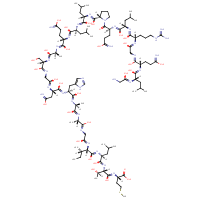Structure InformationCompound Identification

SMILES

CC[C@H](C)[C@H](NC(=O)CNC(=O)[C@H](C)NC(=O)[C@H](C)NC(=O)[C@H](CC1=CNC=N1)NC(=O)[C@H](CC(N)=O)NC(=O)CNC(=O)[C@H](CO)NC(=O)[C@H](C)NC(=O)[C@H](CCC(N)=O)NC(=O)[C@H](CC(C)C)NC(=O)[C@H](CC(C)C)NC(=O)[C@@H]1CCCN1C(=O)[C@H](CCC(N)=O)NC(=O)[C@H](CC(C)C)NC(=O)[C@H](CCCN=C(N)N)NC(=O)CNC(=O)[C@H](CCC(N)=O)NC(=O)[C@H](CC(C)C)NC(=O)CN)C(=O)N[C@@H](CC(C)C)C(=O)N[C@@H]([C@@H](C)O)C(=O)N[C@@H](CCSC)C(O)=O

InChIKey

InChIKey=ZIKKYLJVXBIABZ-DAUJWZHGSA-N

Formula

C101H172N32O30S

Mass

2346.74

### Export to:

Taxonomic Classification

Taxonomy Tree

Kingdom

Organic compounds

Superclass

Organic Polymers

Class

Polypeptides

Subclass

Not available

Intermediate Tree Nodes

Not available

Direct Parent

Polypeptides

Alternative Parents

Molecular Framework

Aromatic heteromonocyclic compounds

Substituents

Polypeptide - Alpha peptide - Histidine or derivatives - Glutamine or derivatives - Methionine or derivatives - Asparagine or derivatives - Leucine or derivatives - Isoleucine or derivatives - N-acyl-alpha amino acid or derivatives - N-acyl-alpha-amino acid - N-acyl-l-alpha-amino acid - Proline or derivatives - Serine or derivatives - Alpha-amino acid amide - Alanine or derivatives - Alpha-amino acid or derivatives - N-substituted-alpha-amino acid - Pyrrolidine carboxylic acid or derivatives - Imidazolyl carboxylic acid derivative - Pyrrolidine-2-carboxamide - N-acylpyrrolidine - Hydroxy fatty acid - Thia fatty acid - N-acyl-amine - Fatty acyl - Fatty amide - Azole - Imidazole - Heteroaromatic compound - Pyrrolidine - Tertiary carboxylic acid amide - Guanidine - Amino acid - Carboxamide group - Amino acid or derivatives - Secondary alcohol - Secondary carboxylic acid amide - Primary carboxylic acid amide - Carboxylic acid derivative - Carboxylic acid - Organic 1,3-dipolar compound - Dialkylthioether - Monocarboxylic acid or derivatives - Propargyl-type 1,3-dipolar organic compound - Azacycle - Thioether - Organoheterocyclic compound - Sulfenyl compound - Carboximidamide - Primary amine - Hydrocarbon derivative - Organic nitrogen compound - Organic oxygen compound - Primary aliphatic amine - Organonitrogen compound - Carbonyl group - Alcohol - Organooxygen compound - Organic oxide - Organosulfur compound - Primary alcohol - Amine - Aromatic heteromonocyclic compound

Description

This compound belongs to the class of organic compounds known as polypeptides. These are peptides containing ten or more amino acid residues.

External Descriptors

Not available